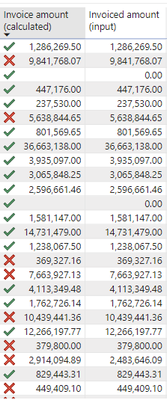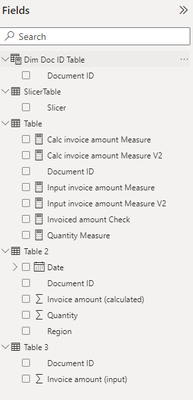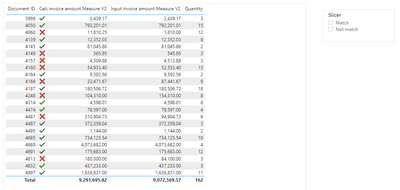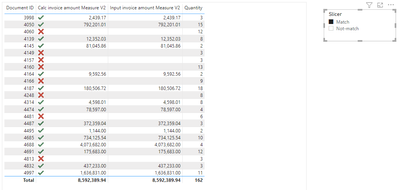cancel
Showing results for
Did you mean:

Fabric is Generally Available. Browse Fabric Presentations. Work towards your Fabric certification with the Cloud Skills Challenge.Anonymous
Not applicable

## Compare numerical values in two columns and flag if not same

Hello,

I have the following table visualization and i am trying to compare the values in both columns and if they do not match, then flag the column on the left. I have created a measure for this purpose, but due to some strange (probably long decimal?) differences, the similar values do not always match. Can this be enforced so that if the whole number values match, then it should be flagged as 1 otherwise 0? Any help is much appreciated!

Here is my measure:

``````Invoiced amount Check =
IF ( [Invoiced amount (calculated)] <> [Invoiced amount (input)], 1, 0 )``````1 ACCEPTED SOLUTIONSuper User

Hi,

Please try the below if it works.

``````Invoiced amount Check =
IF (
ROUND ( [Invoiced amount (calculated)], 0 )
<> ROUND ( [Invoiced amount (input)], 0 ),
1,
0
)
``````

If this post helps, then please consider accepting it as the solution to help other members find it faster, and give a big thumbs up.

9 REPLIES 9Super User

Hi,

Please try the below if it works.

``````Invoiced amount Check =
IF (
ROUND ( [Invoiced amount (calculated)], 0 )
<> ROUND ( [Invoiced amount (input)], 0 ),
1,
0
)
``````

If this post helps, then please consider accepting it as the solution to help other members find it faster, and give a big thumbs up.Anonymous
Not applicable

@Jihwan_Kim Thanks for your reply! Is it possible to implement the measure above into a slicer to filter the rows of the table? For example, a slicer with the following 2 options:

1. Show matching values (this would show only the table rows with the green tick)

2. Show non-matching values (this would show only the table rows with the red cross)Super User

Hi,

I think it is possible.

If you can share your pbix file's link here, and then I can try to look into it to come up with a solution.

Thanks.

If this post helps, then please consider accepting it as the solution to help other members find it faster, and give a big thumbs up.Anonymous
Not applicableSuper User

Hi,

Please kindly check the attached pbix file.

I suggest having a disconnected slicer table like the attached, and create measures that corresponds with the slicer selection. (V2 measures in the attached pbix file).

Thank you.

If this post helps, then please consider accepting it as the solution to help other members find it faster, and give a big thumbs up.Anonymous
Not applicable

@Jihwan_Kim The slicer works but it is limited to only columns in the same table. I have added other tables with extra columns and made some relationships between the tables. So, i have updated the measures accordingly with the different table/column names.

I would like to include another column in the filtered results but when selecting the slicer options, it is only filtering in static mode - by keeping the same number of rows. Is it possible to fix this, so that it shows only the match or non-match list?The updated measures:

``````Calc invoice amount Measure V2 =
SWITCH (
SELECTEDVALUE ( SlicerTable[Slicer] ),
"Match",
CALCULATE (
SUM ( 'Table 2'[Invoice amount (calculated)] ),
FILTER (
SUMMARIZE ( 'Table', 'Table'[Document ID] ),
[Invoiced amount Check] = 0
)
),
"Not-match",
CALCULATE (
SUM ( 'Table 2'[Invoice amount (calculated)] ),
FILTER (
SUMMARIZE ( 'Table', 'Table'[Document ID] ),
[Invoiced amount Check] = 1
)
),
SUM ( 'Table 2'[Invoice amount (calculated)] )
)``````

``````Input invoice amount Measure V2 =
SWITCH (
SELECTEDVALUE ( SlicerTable[Slicer] ),
"Match",
CALCULATE (
[Input invoice amount Measure],
FILTER (
SUMMARIZE ( 'Table', 'Table'[Document ID] ),
[Invoiced amount Check] = 0
)
),
"Not-match",
CALCULATE (
[Input invoice amount Measure],
FILTER (
SUMMARIZE ( 'Table', 'Table'[Document ID] ),
[Invoiced amount Check] = 1
)
),
[Input invoice amount Measure]
)``````

With no filter applied:After filter selection:Super User

Hi,

Sorry that I do not understand.

Could you please check the attached file if it is what you are looking for? I tried to create a explicit measure for Quantity, instead of using Quantity Column itself.

Thank you.

If this post helps, then please consider accepting it as the solution to help other members find it faster, and give a big thumbs up.Anonymous
Not applicable

@Jihwan_Kim Thanks! It works. I have tried to add the "Region" field as a new column to the table and adapted the measure but i get an error. Can you please suggest a solution?

``````Region Measure V2 =
SWITCH (
SELECTEDVALUE ( SlicerTable[Slicer] ),
"Match",
CALCULATE (
SUM ( 'Table 2'[Region] ),
FILTER ( SUMMARIZE ( 'Table', 'Table'[Document ID] ),
[Invoiced amount Check] = 0
)
),
"Not-match",
CALCULATE (
SUM ( 'Table 2'[Region] ),
FILTER (
SUMMARIZE ( 'Table', 'Table'[Document ID] ),
[Invoiced amount Check] = 1
)
),
SUM ( 'Table 2'[Region] )
)``````I changed the 2 "SUM" to "COUNT" in the measure but it still does not work.Super User

Hi,

Do you want to add Region column from Table2?

I added the column like the attached file, but sorry that I could not understand the measure you wrote. May I ask what do you want to show by writing a Region measure V2 ?

If this post helps, then please consider accepting it as the solution to help other members find it faster, and give a big thumbs up.Announcements#### Power BI Monthly Update - November 2023

Check out the November 2023 Power BI update to learn about new features.#### Fabric Community News unified experience

Read the latest Fabric Community announcements, including updates on Power BI, Synapse, Data Factory and Data Activator.#### The largest Power BI and Fabric virtual conference

130+ sessions, 130+ speakers, Product managers, MVPs, and experts. All about Power BI and Fabric. Attend online or watch the recordings.Top Solution Authors
Top Kudoed Authors
Users online (3,581)